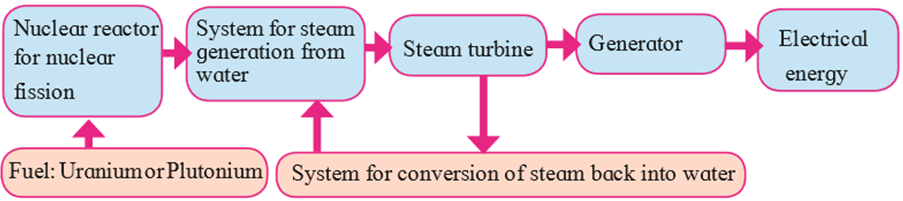### Explain with diagram step-by-step energy conversion in Nuclear Power Plant:

Question 14.

Explain with diagram step-by-step energy conversion in

Nuclear Power Plant:

Answer:

Here nuclear energy is converted into thermal energy, thermal energy is converted into kinetic energy of steam, kinetic energy of steam is converted into kinetic energy of turbine and finally the kinetic energy of the turbine is converted into electrical energy .The step-by-step transformation of energy is shown in the diagram below: